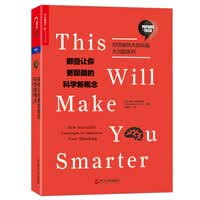预测性编码《那些让你更聪明的科学新概念》封面

- 阅读剩余部分 -

得到APP《混沌——开创新科学》讲书稿《混沌——开创新科学》20周年版封面“蝴蝶效应”的一个经典描述，“一只蝴蝶在巴西轻拍翅膀，可以导致一个月后美国的一场龙卷风”。

- 阅读剩余部分 -

文化吸引子《那些让你更聪明的科学新概念》封面

- 阅读剩余部分 -

噪声RC电路\begin{equation} \begin{split} \langle \xi(t)\rangle = &0\\ \langle \xi(t)\xi(t')\rangle = &2B\delta(t-t') \end{split} \label{noisesource} \end{equation}

- 阅读剩余部分 -

爱因斯坦的奥卡姆剃刀《那些让你更聪明的科学新概念》封面

“简单”无疑非常重要，但过于简化未必就是最好。爱因斯坦的奥卡姆剃刀，这个概念可以帮助我们理清简单与复杂之间的关系，帮助我们理解各种复杂的事情。

- 阅读剩余部分 -

带电平面之间的相互作用（无外加盐）- 阅读剩余部分 -

清华大学教授安宇：为什么大学生上课不听课，听课听不会？- 阅读剩余部分 -

温伯格：科学与宗教之间的紧张关系的4个来源- 阅读剩余部分 -

令人哇凉哇凉的测验

1. 如下图所示，长为$L$的带电棒左、右半段分别带有负电$-Q$和正电$Q$，且均匀带电，求带电棒中垂线上距离棒$a$处的电场强度。1. 如下图所示，半径为$R_1$的均匀带电球体的电荷体密度为$\rho$，球外有半径分别为$R_2$和$R_3$的导体球壳，计算电场强度和电势的分布。计算体系中总的静电能。1. 如下图所示，圆柱形电容器，长为$l$，中间充满两层电介质，相对电容率分别为$\varepsilon_1$和$\varepsilon_2$，内外导体圆筒半径分别为$R_1$和$R_3$，且$l\gg R_3$，电介质分界面半径为$R_2$，求此电容器电容。（提示：根据有电介质时的高斯定理求出电位移和电场的分布，计算出内外导体圆筒的电势差，进而得电容。）